# Row_Number Function in SQL Server

## Row_Number Function in SQL Server with Examples

In this article, I am going to discuss Row_Number Function in SQL Server with Examples. Please read our previous article where we discussed the OVER Clause in SQL Server before proceeding to this article. At the end of this article, you will understand what exactly the ROW_NUMBER function is and when and how to use the Row Number function in SQL Server. As part of this article, I am going to discuss the following pointers.

1. What is ROW_NUMBER Function in SQL Server?
2. Understanding the importance of PARTITION BY and ORDER BY Clause in ROW_NUMBER Function.
3. How to use the ROW_NUMBER Function with and without the PARTITION BY clause?
4. Multiple examples to understand the Row_Number function.
5. Finally, one real-time example of the ROW_NUMBER Function.
##### ROW_NUMBER Function in SQL Server:

The Row Number function was introduced in SQL Server 2005. The ROW_NUMBER function is basically used when you want to return a sequential number starting from 1.

The ROW_NUMBER() is a built-in function in SQL Server that assigns a sequential integer number to each row within a partition of a result set. The row number always starts with 1 for the first row in each partition and then increases by 1 for the next row onwards in each partition. The syntax to use the ROW_NUMBER function is given below.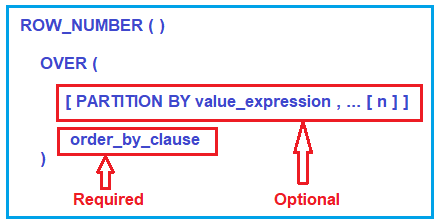###### PARTITION BY value_expression:

This is optional. The PARTITION BY clause is used to divide the result set that is produced by the FROM clause into partitions and then the ROW_NUMBER function is applied to each partition. Here, the value_expression specifies the column name (s) using which the result set is going to be partitioned. As it is optional, if you did not specify the PARTITION BY clause, then the ROW_NUMBER function will treat all the rows of the query as a single group.

##### Order_by_clause:

It is required. The ORDER BY clause is basically used to define the sequence in which each row is going to assign its unique ROW_NUMBER.

Note: If you did not use the PARTITION BY clause with the ROW_NUMBER function, then the result set will consider as a single partition.

##### Example: Row_Number Function in SQL Server

Let us see some examples to understand the ROW NUMBER function in SQL Server. We are going to use the following Employee table.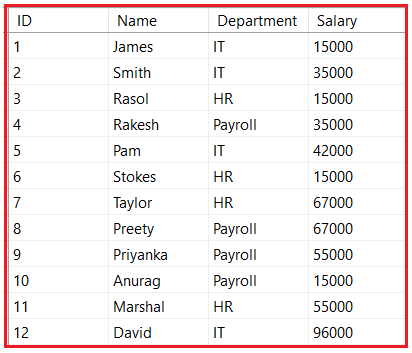Please use the following SQL Script to create and populate the Employees table with the required data.

```CREATE TABLE Employees
(
ID INT,
Name VARCHAR(50),
Department VARCHAR(50),
Salary int
)
Go

INSERT INTO Employees Values (1, 'James', 'IT', 15000)
INSERT INTO Employees Values (2, 'Smith', 'IT', 35000)
INSERT INTO Employees Values (3, 'Rasol', 'HR', 15000)
INSERT INTO Employees Values (4, 'Rakesh', 'Payroll', 35000)
INSERT INTO Employees Values (5, 'Pam', 'IT', 42000)
INSERT INTO Employees Values (6, 'Stokes', 'HR', 15000)
INSERT INTO Employees Values (7, 'Taylor', 'HR', 67000)
INSERT INTO Employees Values (8, 'Preety', 'Payroll', 67000)
INSERT INTO Employees Values (9, 'Priyanka', 'Payroll', 55000)
INSERT INTO Employees Values (10, 'Anurag', 'Payroll', 15000)
INSERT INTO Employees Values (11, 'Marshal', 'HR', 55000)
INSERT INTO Employees Values (12, 'David', 'IT', 96000)
```
##### ROW_NUMBER Function without PARTITION BY:

Let us see an example of the ROW_NUMBER function without using the PARTITION BY Clause. As we already discussed if we did not specify the PARTITION BY Clause, then the ROW_NUMBER function will treat the whole result set as a single group. As a result, the ROW_NUMBER function will provide a consecutive sequence numbering for all the rows present in the result set based on the column(s) specified in the order by clause. The following is an example of the ROW_NUMBER function without using the PARTITION BY clause.

SELECT Name, Department, Salary,
ROW_NUMBER() OVER (ORDER BY Department) AS RowNumber
FROM Employees

Once you execute the above query, you will get the below output. As you can see in the below output, there will be no partition and hence all the rows are assigned with consecutive sequence numbering starting from 1 to 12.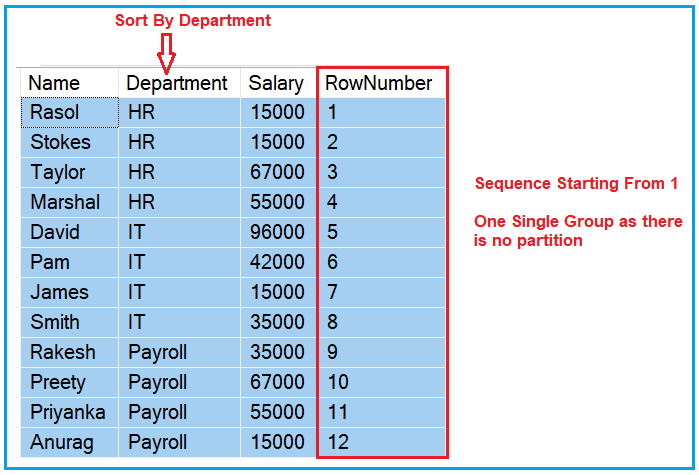Note: If you did not specify the ORDER BY clause then you will get the error stating “The function ‘ROW_NUMBER’ must have an OVER clause with ORDER BY”.

##### Row_Number Function with PARTITION BY Clause:

Let us see an example of the ROW_NUMBER function using the PARTITION BY Clause. When you specify the PARTITION BY Clause, then the data is partitioned based on the column you specify in the Partition BY clause. Please have a look at the following image to understand this better. As you can see we have specified Department in the Partition By clause and Name in the Order By clause.

In our example, we have three departments. So, the Partition By Clause will divide all the rows into three partitions or you can say three groups. Then in each group or in each partition, the data is sorted based on the Name column. The ROW_NUMBER function then gives a unique sequence to each partition starting from 1. As we have three partitions, each partition will start from 1 that which you can see in the below image.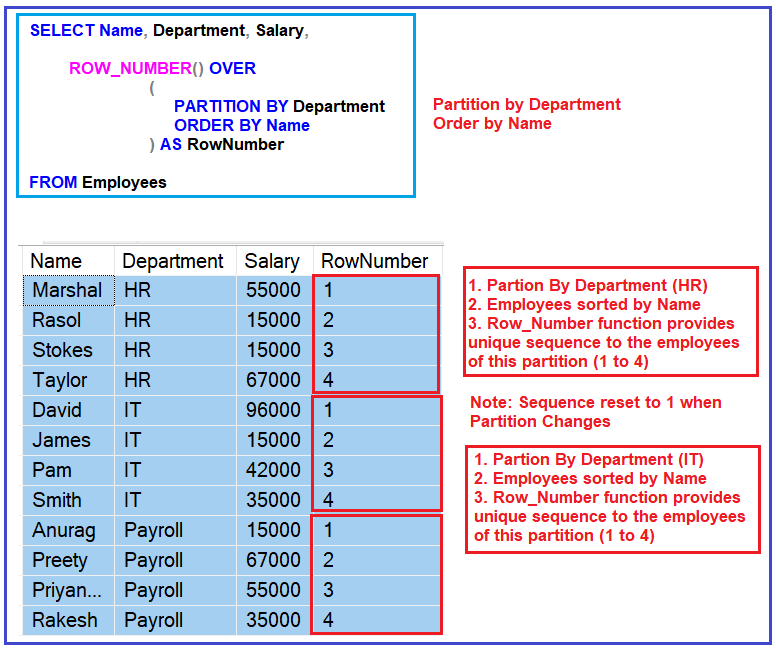Now execute the following code and you will get the output as we discussed in the previous image.

```SELECT Name, Department, Salary,
ROW_NUMBER() OVER
(
PARTITION BY Department
ORDER BY Name
) AS RowNumber
FROM Employees
```

Note: When the partition changes the row number is reset to 1. So, basically whenever you want to give a unique number on the fly to the rows in the result set, then you need to use the ROW_NUMBER function.

##### Real-time Example of ROW_NUMBER function in SQL Server:

Let us see one real-time example of the ROW_NUMER function in SQL Server. To understand this first delete all the records which are there in the Employees table by executing the following SQL query.

TRUNCATE TABLE Employees

Once you delete all the data from the Employees table then please insert the following records into the Employees table.

```INSERT INTO Employees Values (1, 'James', 'IT', 15000)
INSERT INTO Employees Values (1, 'James', 'IT', 15000)
INSERT INTO Employees Values (2, 'Rasol', 'HR', 15000)
INSERT INTO Employees Values (2, 'Rasol', 'HR', 15000)
INSERT INTO Employees Values (2, 'Rasol', 'HR', 15000)
INSERT INTO Employees Values (3, 'Stokes', 'HR', 15000)
INSERT INTO Employees Values (3, 'Stokes', 'HR', 15000)
INSERT INTO Employees Values (3, 'Stokes', 'HR', 15000)
INSERT INTO Employees Values (3, 'Stokes', 'HR', 15000)
```

Now, intentionally we have inserted some duplicate data into the Employees table as shown below.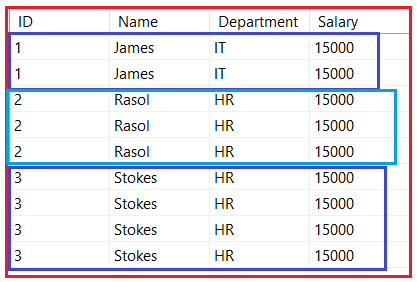Now we need to delete the duplicate records from the Employees table. Once you delete the duplicate data, then your table should have the following record.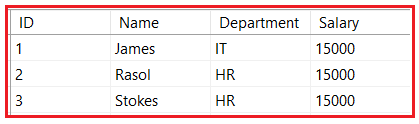The following SQL query will delete the duplicate records from the Employees table. Here, the PARTITION BY clause divides the query result set into partitions based on the ID values. That means it will create multiple partitions based on the ID values and give sequential integer numbers starting from 1 for each partition. Then here the DELETE statements will delete the records from each partition where the RowNumber is greater than 1. It means it will keep only one record from each partition and delete the rest of all.

WITH DeleteDuplicateCTE AS
(
SELECT *, ROW_NUMBER() OVER(PARTITION BY ID ORDER BY ID) AS RowNumber
FROM Employees
)
DELETE FROM DeleteDuplicateCTE WHERE RowNumber > 1

Once you execute the above SQL query, now verify the Employees table and you can see the duplicates records are deleted. So, in short, the ROW_NUMBER function Returns an increasing unique number for each row starting from 1, even if there are duplicates.

In the next article, I am going to discuss the RANK and DENSE_RANK function in SQL Server with examples. Here, in this article, I try to explain the ROW_NUMBER Function in SQL Server with Examples. I hope now you understand the need and use of the ROW_NUMBER function in SQL Server with Examples.

### 2 thoughts on “Row_Number Function in SQL Server”

1.Article is so nice to understand.

2.Valuable information